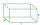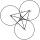# Triangular prism

Calculate the volume and surface area of a triangular prism if it is given:
a = 6.8 dm. ..Va = 4 dm. (base edge length and base triangle height length) ... ... .v = 23 dm (body height)

Result

V =  312.8 dm3
S =  496.4 dm2

#### Solution:Leave us a comment of example and its solution (i.e. if it is still somewhat unclear...):

Showing 0 comments:Be the first to comment!#### To solve this example are needed these knowledge from mathematics:

Tip: Our volume units converter will help you with converion of volume units. See also our trigonometric triangle calculator.

## Next similar examples:

1. Triangular prismCalculate the surface area and volume of a triangular prism, base right triangle if a = 3 cm, b = 4 cm, c = 5 cm and height of prism h=12 cm.
2. Triangular prismCalculate the volume of a triangular prism 10 cm high, the base of which is an equilateral triangle with dimensions a = 5 cm and height va = 4,3 cm
3. 3s prismIt is given a regular perpendicular triangular prism with a height 19.0 cm and a base edge length 7.1 cm. Calculate the volume of the prism.
4. Triangular prismThe perpendicular triangular prism is a right triangle with a 5 cm leg. The content of the largest wall of the prism is 130 cm2 and the body height is 10 cm. Calculate the body volume.
5. Regular triangular pyramidCalculate the volume and surface area of the regular triangular pyramid and the height of the pyramid is 12 centimeters, the bottom edge has 4 centimeters and the height of the side wall is 12 centimeters
6. Prism - eq triangleCalculate the volume and surface of the prism with the base of an equilateral triangle with side a = 4cm and the body height is 6cm.
7. PrismCalculate the surface area and volume of a prism with a body height h = 10 cm and its base has shape of a rhomboid with sides a = 5.8 cm, b = 3 cm and the distance of its two longer sides is w = 2.4 cm.
8. Square prismCalculate the volume of a foursided prism 2 dm high, the base is a trapezoid with bases 12 cm, 6 cm, height of 4 cm and 5 cm long arms.
9. Scale factorA prism with a volume of 1458 mm3 is scaled down to a volume of 16mm3. What is the scale factor in fraction form?
10. Cylinder surface areaVolume of a cylinder whose height is equal to the radius of the base is 678.5 dm3. Calculate its surface area.
11. Truncated coneA truncated cone has a bases radiuses 40 cm and 10 cm and a height of 25 cm. Calculate its surface area and volume.
12. Cone area and sideCalculate the surface area and volume of a rotating cone with a height of 1.25 dm and 17,8dm side.
13. Cube volumeThe cube has a surface of 384 cm2. Calculate its volume.
14. Metal railsDad needs to improve the edges of the wooden boxes - to be reinforced with metal rails. How many cms of rails will he need if the box has the shape of a prism with the length of the edges 70cm 70cm and 120cm?
15. Fit ballWhat is the size of the surface of Gymball (FIT - ball) with a diameter of 65 cm?
16. Center traverseIt is true that the middle traverse bisects the triangle?
17. CirclesThree circles of radius 95 cm 78 cm and 64 cm is mutually tangent. What is the perimeter of the triangle whose vertices are centers of the circles?